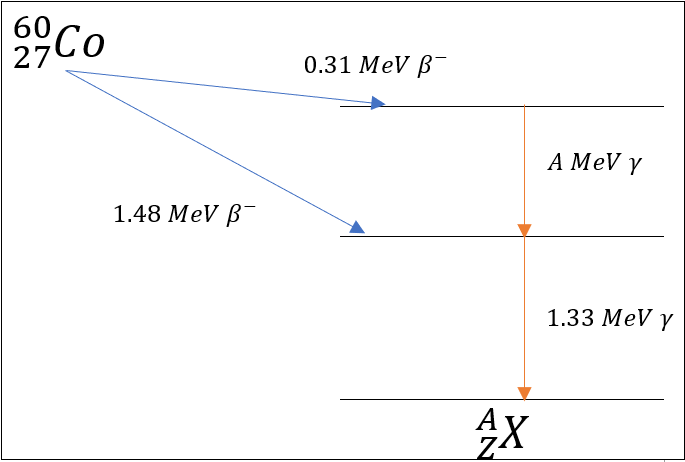# Nuclear Reactions

A nuclear reaction occurs between nuclides and fundamental particles to produce different nuclides. This is different from a chemical reaction where electrons are shared/transfered between elements and compounds are changed. Nuclear reactions release significantly more enegy and result in a change in mass between intial reactants and products.

When a nuclide is unstable (radioactive) is can decay by various nuclear processes to a lower (stable) energy state. Some examples are shown below.

 Decay Mode General Equation Description Alpha $${^A_Z}X \rightarrow {^{A-4}_{Z-2}}X^{'} + {^4_2}\alpha$$ Heavy atoms release a He particle. Beta (-) $${^A_Z}X \rightarrow {^A_{Z+1}}X^{'} + \ce{^0_-1\beta}$$ A neutron is converted into a proton and an electron is released. Beta (+) $${^A_Z}X \rightarrow {^A_{Z-1}}X^{'} + \ce{^0_+1\beta}$$ A proton is converted into a neutron and a positron is released. Proton Emission $${^A_Z}X \rightarrow {^{A-1}_{Z-1}}X^{'} + \ce{^1_1p}$$ A proton is ejected. Neutron Emission $${^A_Z}X \rightarrow {^{A-1}_{Z}X} + \ce{^1_0n}$$ A neutron is ejected. Internal Conversion $${^A_Z}X \rightarrow {^A_ZX} + \ce{^0_-1e}$$ Electron is ejected. Gamma $${^A_Z}X \rightarrow {^A_ZX} + \gamma$$ Energy is released but the nuclide does not change (isomer). Spontaneous Fission $$X \rightarrow Y + Z \; ...$$ A nuclide splits into two or more smaller nuclie and other particles.

A radioactive nuclide may have different decay modes. It may also decay to another unstable daughter nuclide which subsequently decays. A decay scheme is a visual representation of the decay modes for a nuclide.

The decay scheme for Co-60 is shown below. What is the daughter nuclide and energy of the missing gamma decay?Other nuclear reactions invovle the interaction between nuclides or particles. Some examples are shown below.

 Reaction Equation Description Radiative Capture $$(n,\gamma)$$ Absorption of a neutron and release of a gamma. Particle Emission $$(n,\alpha), (n,p), (n,2n)$$ Absorption of a neutron and release of a particle. Induced Fission $$(n,f)$$ Absorption of a neutron, splitting into multiple nuclides and particles. Fusion $$A + B + \; ... = C + \; ...$$ Combination of multiple nuclides and particles.

What is the result of the following equation: $$^{10}B(n,\alpha)$$